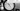# Moment.js - How to perform date relatedd arithmetic in javascript/NodeJs

May 14, 2020## Introduction

In your backend and frontend projects, you always need to deal with dates. Some examples are:

• Get date of same day next month
• Get date of current minus 10 days
• Extend date of your subscription validity

Writing plain javascript code is error prone, you need to be super sure of what you are doing. MomentJs is your savior. It is much popular in this domain. It provides lot of handy functions/methods which are very useful in date related arithmetics.

Lets take a look at some of common usages:

## Get current date

``````const moment = require('moment');
const currentDate = moment();
``````

## Get date difference in terms of days/hours/minutes

``````const moment = require('moment');

const now = moment(new Date().toISOString());
console.log(now);
var dates = [
moment('2017-12-30T11:36:51.992Z'),
moment('2017-12-29T11:36:51.992Z'),
moment('2017-12-28T11:36:51.992Z'),
moment('2017-12-27T11:36:51.992Z'),
moment('2017-12-26T11:36:51.992Z'),
moment('2017-12-25T11:36:51.992Z'),
];

for(let i=0; i<dates.length; i++) {
let dt = dates[i];
console.log('date', dt);
console.log('Days', now.diff(dt, 'days'));
console.log('hours', now.diff(dt, 'hours'));
console.log('minutes', now.diff(dt, 'minutes'));

}
``````

## Add ‘N’ days to current date

Add 15 days to current date.

``````const moment = require('moment');

const now = moment();
console.log(now);

console.log(now);

// Output
// moment("2020-05-20T14:01:29.933")
// moment("2020-06-04T14:01:29.933")``````

Note above that by calling add function, the object now is updated. You can assign it to another variable as well. But, both the objects will have same value. See below section for not updating calling object.

``````const moment = require('moment');

const now = moment();
console.log(now);

console.log(now);
console.log(after);

// Output
// moment("2020-05-20T14:04:57.000")
// moment("2020-06-04T14:04:57.000")
// moment("2020-06-04T14:04:57.000")``````

Similarly you can add months and years to a date. By using string months, years.

## Get Date of next month, same day

Note: Its not like adding 30 days to current date.

``````const moment = require('moment');

const now = moment();
console.log(now);

//next month, same date
console.log(after);

//next month, same date
console.log(after);

//next month, same date
console.log(after);

// Output
// moment("2020-05-20T14:06:35.099")
// moment("2020-06-20T14:06:35.099")
// moment("2020-07-20T14:06:35.099")
// moment("2020-08-20T14:06:35.099")``````

## Get date of last month

``````
const moment = require('moment');

const now = moment();
console.log(now);

//next month, same date
let after = now.subtract(1, 'months');
console.log(after);

// Output
// moment("2020-05-20T14:07:42.037")
// moment("2020-04-20T14:07:42.037")``````

## Get date of next week

``````const moment = require('moment');

const now = moment();

//next month, same date
console.log(now);
console.log(after);

// Output
// moment("2020-05-20T14:11:27.766")
// moment("2020-05-27T14:11:27.766")``````

## Very Important - How to not update original object while adding/subtracting dates

If you notice above, that the value of object now changed by calling add/subtract over the calling object. To avoid this, use clone()

``````const moment = require('moment');

const now = moment();
console.log(now);

//next month, same date
let after = now.clone().subtract(1, 'months');
console.log(now);
console.log(after);

// Output
moment("2020-05-20T14:10:10.061")
moment("2020-05-20T14:10:10.061")
moment("2020-04-20T14:10:10.061")``````